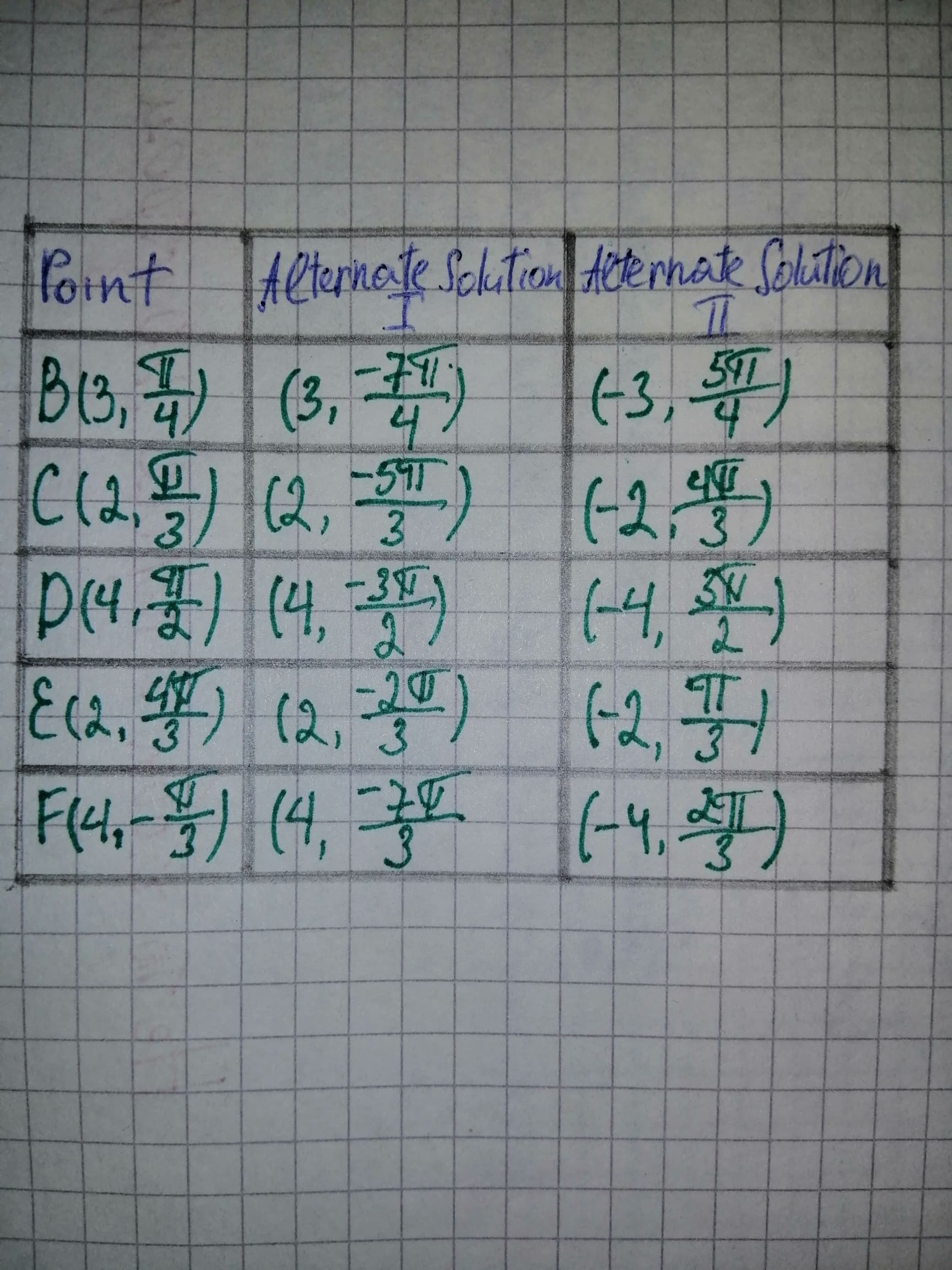# Thealternate sets of representations for the points A-F from the Figure.Phoebe 2020-11-16 Answered
Thealternate sets of representations for the points A-F from the Figure.
You can still ask an expert for help

• Questions are typically answered in as fast as 30 minutes

Solve your problem for the price of one coffee

• Math expert for every subject
• Pay only if we can solve itmhalmantus

Consider the point A, it lies on the angle $\theta =\frac{\pi }{6}$ and the distance AO is 4 units where O is the origin.
Therefore, the point A is represented by $\left(4,\frac{\pi }{6}\right)$ in polar coordinate system.
Subtract $2\pi$ from the angle to get the alternate solution.
Therefore, the point $A\left(4,\frac{\pi }{6}\right)$ can also be represented by $\left(4,\frac{\pi }{6}-2\pi \right)=\left(4,-\frac{11\pi }{6}\right).$
Change the radial coordinate to -4 and add pi from the angle to obtain the other alternate solution. Thus, the point P $\left(4,\frac{\pi }{6}\right)$ can be given by $\left(-4,\frac{\pi }{6}+\pi \right)=\left(-4,\frac{7\pi }{6}\right).$
Similarly, calculate the alternate representations for the points B to F and obtain the results as shown in the below table.Hence, Alternate representation for the points A-F listed.